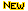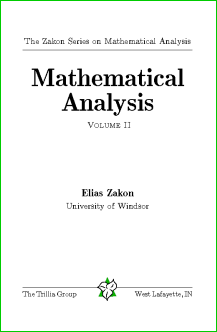Processing ......FreeComputerBooks.com Links to Free Computer, Mathematics, Technical Books all over the World

Mathematical Analysis IITop Free Java Books - 100% Free or Open Source!
• Title Mathematical Analysis II
• Author(s) Elias Zakon
• Publisher: Trillia Group, 2009.
• Hardcover 424 pages
• eBook PDF Files
• Language: English
• ISBN-10: 1-931705-03-8
• ISBN-13: N/ABook Description

This final text in the Zakon Series on Mathematics Analysis follows the release of the author's Basic Concepts of Mathematics and Mathematical Analysis I and completes the material on Real Analysis that is the foundation for later courses in functional analysis, harmonic analysis, probability theory, etc.

The first chapter extends calculus to n-dimensional Euclidean space and, more generally, Banach spaces, covering the inverse function theorem, the implicit function theorem, Taylor expansions, etc. Some basic theorems in functional analysis, including the open mapping theorem and the Banach-Steinhaus uniform boundedness principle, are also proved.

The text then moves to measure theory, with a complete discussion of outer measures, Lebesgue measure, Lebesgue-Stieltjes measures, and differentiation of set functions. The discussion of measurable functions and integration in the following chapter follows an innovative approach, carefully choosing one of the equivalent definitions of measurable functions that allows the most intuitive development of the material. Fubini's theorem, the Radon-Nikodym theorem, and the basic convergence theorems (Fatou's lemma, the monotone convergence theorem, dominated convergence theorem) are covered.

Finally, a chapter relates antidifferentiation to Lebesgue theory, Cauchy integrals, and convergence of parametrized integrals.

Nearly 500 exercises allow students to develop their skills in the area.

 :All CategoriesTop BooksRecent BooksMiscellaneous BooksComputer LanguagesComputer ScienceData Science/DatabasesElectrical EngineeringJava and Java EE (J2EE)Linux and UnixMathematicsMicrosoft and .NETMobile ComputingNetworking and CommunicationsSoftware EngineeringSpecial TopicsWeb Programming